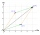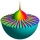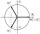# Complex numbers + addition - math problems

#### Number of problems found: 5

• An Argand diagramFind the sum z1+z2 and difference z1-z2 and z2-z1 of z1=5+2i and z2=2+3i by an Argand diagram.
• Linear combination of complexIf z1=5+3i and z2=4-2i, write the following in the form a+bi a) 4z1+6z2 b) z1*z2
• BearingA plane flew 50 km on a bearing 63°20' and the flew on a bearing 153°20' for 140km. Find the distance between the starting point and the ending point.
• Imaginary numbersFind two imaginary numbers whose sum is a real number. How are the two imaginary numbers related? What is its sum?
• De Moivre's formulaThere are two distinct complex numbers z, such that z3 is equal to 1 and z is not equal to 1. Calculate the sum of these two numbers.

We apologize, but in this category are not a lot of examples.
Do you have an exciting math question or word problem that you can't solve? Ask a question or post a math problem, and we can try to solve it.

We will send a solution to your e-mail address. Solved examples are also published here. Please enter the e-mail correctly and check whether you don't have a full mailbox.

Try our complex numbers calculator. Complex numbers - math problems. Addition Problems.